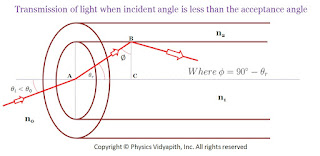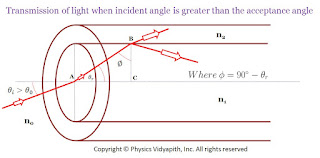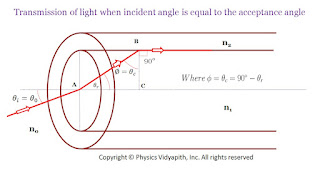### Numerical Aperture and Acceptance Angle of the Optical Fibre

Angle of Acceptance →

The angle of incident of light on the core for which the angle of incidence on the core-cladding interface equals the critical angle is known as the "Angle of Acceptance".Transmission of light when the incident angle is less than the acceptance angle

For $\theta_{i}>\theta_{0}$, the angle of incidence on the core-cladding interface is less than the critical angle due to which part of incident light is transmitted into cladding as shown in the figure belowTransmission of light when the incident angle is greater than the acceptance angle
Hence the light will be transmitted into cladding for angles of incidence on the core which are less than the acceptance angle.

The acceptance angle is the maximum angle of incidence on the core for which total internal reflection takes place inside the core.

The light entering the core in a cone of semi-vertical angle $\theta_{0}$ is transmitted in the core through total internal reflections. This cone is known as the acceptance cone.

Numerical Aperture

"The sine of the angle of acceptance of the optical fibre is known as the numerical aperture of optical fibre."

The numerical aperture determines the light-gathering ability of the fibre. It measures the amount of light that can be accepted by a fibre. The numerical aperture depends upon the refractive index of the core and cladding material and does not depend on the physical dimension of the fibre. It is a dimensionless quantity that is less than unity. The value of the numerical aperture range from $0.13$ to $0.15$. A large numerical aperture implies that a fibre accepts a large amount of light from the source. It varies due to variations of refractive index in the core and it has zero value after the core-cladding boundary. The number of propagation modes to multimode graded-index fibre depends upon the parameter of numerical aperture and hence upon the relative refractive index difference $\Delta n$

Derivation of Angle of Acceptance and Numerical Aperture

Let us consider, step-index optical fibre into which light is launched from one end at angle $\theta_{i}$. Let the refractive index of core and cladding is $n_{1}$ and $n_{2}$.Transmission of light when the incident angle is equal to the acceptance angle
Let us consider that light rays enter the fibre at an angle $\theta_{i}$ to the axis of the fibre refracted at an angle $\theta_{r}$ and strike the core-cladding interface at an angle $\phi$.

When ray incident at point $A$ on the core then According to Snell's law

$\frac{sin \theta_{0}}{sin \theta_{r}}= \frac{n_{1}}{n_{0}}$

Where $n_{0}$ → refractive index of air and vacuum

$sin\theta_{0}=\frac{n_{1}}{n_{0}} sin \theta_{r} \qquad(1)$

Now the refracted ray incident at point $B$ at the interface of core and cladding. So for critical angle condition

$n_{1}\: sin\phi=n_{2} \: sin90^{0}$

$n_{1}\: sin(90-\theta_{r})=n_{2} \: sin90^{0} \qquad (\because \phi=90-\theta_{r})$

$n_{1}\: cos \theta_{r}=n_{2}$

$cos\theta_{r}=\frac{n_{2}}{n_{1}} \qquad(2)$

$sin\theta_{r}=\sqrt{1-cos^{2}\theta_{r}}$

Now substitue the value of $cos\theta_{r}$ from equation$(2)$ to above equation then we get

$sin\theta_{r}=\sqrt{1- \left ( \frac{n_{2}}{n_{1}} \right)^{2}}$

$sin\theta_{r}=\frac{1}{n_{1}}\sqrt{n_{1}^{2}- n_{2}^{2}}$

Now substitue the value of $sin\theta_{r}$ in equation$(1)$ then we get

$sin\theta_{0}=\frac{n_{1}}{n_{0}}\frac{1}{n_{1}}\sqrt{n_{1}^{2}- n_{2}^{2}}$

 $sin\theta_{0}=\frac{\sqrt{n_{1}^{2}- n_{2}^{2}}}{n_{0}}\qquad(3)$

This $sin\theta_{0}$ is known as Numerical Aperture. i.e.

 $N.A.=\frac{\sqrt{n_{1}^{2}- n_{2}^{2}}}{n_{0}}$

If fibre is in the air then $n_{0}=1$ so the above equation can be written as

 $N.A.=\sqrt{n_{1}^{2}- n_{2}^{2}}$

The equation $(3)$ also can be written as

 $\theta_{0}=sin^{-1}\frac{\sqrt{n_{1}^{2}- n_{2}^{2}}}{n_{0}}$

The angle $\theta_{0}$ is known as the Angle of Acceptance.

The light is transmitted through the fibre when

$\theta_{i} < \theta_{0}$

i.e. $sin \theta_{i} < sin \theta_{0}$

 $sin \theta_{i} < N.A.$

The light will be transmitted through the fibre with multiple total internal reflections when the above condition is satisfied.